SSC (English Medium) Class 10th Board ExamMaharashtra State Board
Share

# Balbharati solutions for Class 10th Board Exam Algebra chapter 3 - Arithmetic Progression

## Balbharati Class 10 Mathematics 1 Algebra

#### Balbharati Balbharati Class 10 Mathematics 1 Algebra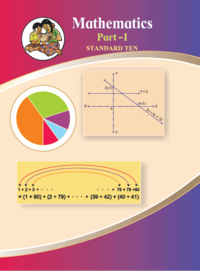## Chapter 3: Arithmetic Progression

#### Chapter 3: Arithmetic Progression solutions [Pages 6 - 62]

Q 1.1 | Page 61

Which of the following sequences are A.P. ? If they are A.P. find the common difference .

2, 4, 6, 8,....

Q 1.2 | Page 61

Which of the following sequences are A.P. ? If they are A.P. find the common difference .

2,$\frac{5}{2}$, 3,$\frac{7}{3}$,......
Q 1.3 | Page 61

Which of the following sequences are A.P. ? If they are A.P. find the common difference .

–10, –6, –2, 2...

Q 1.4 | Page 61

Which of the following sequences are A.P. ? If they are A.P. find the common difference .

0.3, 0.33, 0.333,...

Q 1.5 | Page 61

Which of the following sequences are A.P. ? If they are A.P. find the common difference .

0, –4, –8, –12,...

Q 1.6 | Page 61

Which of the following sequences are A.P. ? If they are A.P. find the common difference .

$- \frac{1}{5}, - \frac{1}{5}, - \frac{1}{5}, . . .$
Q 1.7 | Page 61

Which of the following sequences are A.P. ? If they are A.P. find the common difference .

3,$3 + \sqrt{2}, 3 + 2\sqrt{2}, 3 + 3\sqrt{2}$

Q 1.8 | Page 61

Which of the following sequences are A.P. ? If they are A.P. find the common difference .

127, 132, 137,...

Q 2.1 | Page 61

Write an A.P. whose first term is a and common difference is d in each of the following.

a = 10, d = 5

Q 2.2 | Page 61

Write an A.P. whose first term is a and common difference is d in each of the following.

a = –3, d = 0

Q 2.3 | Page 61

Write an A.P. whose first term is a and common difference is d in each of the following.

a = –7, d = $\frac{1}{2}$

Q 2.4 | Page 61

Write an A.P. whose first term is a and common difference is d in each of the following.

a = –1.25, d = 3

Q 2.5 | Page 61

Write an A.P. whose first term is a and common difference is d in each of the following.

a = 6, d = –3

Q 2.6 | Page 61

Write an A.P. whose first term is a and common difference is d in each of the following.

a = 19, d = –4

Q 3.1 | Page 62

Find the first term and common difference for each of the A.P.

5, 1, –3, –7,...

Q 3.2 | Page 62

Find the first term and common difference for each of the A.P.

0.6, 0.9, 1.2,1.5,...

Q 3.3 | Page 6

Find the first term and common difference for each of the A.P.

127, 135, 143, 151,...

Q 3.4 | Page 62

Find the first term and common difference for each of the A.P.

$\frac{1}{4}, \frac{3}{4}, \frac{5}{4}, \frac{7}{4}, . . . .$

#### Chapter 3: Arithmetic Progression solutions [Pages 62 - 66]

Q 1.1 | Page 66

Write the correct number in the given boxes from the following A. P.

1, 8, 15, 22,...
Here a =Here a =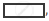t=t2 =t3 =,

t2 – t1 ==t3 – t2 ==∴ d =Q 1.2 | Page 66

Write the correct number in the given boxes from the following A. P.

3, 6, 9, 12,...

Here  t=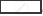t2 =t3 =t4 =,
t2 – t1 =t3 – t2 =∴ d =Q 1.3 | Page 62

Write the correct number in the given boxes from the following A. P.

–3, –8, –13, –18,...

Here  t=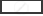t2 =t4 =t1 =,
t2 – t1 =t3 – t2 =∴ a =d =Q 1.4 | Page 66

Write the correct number in the given boxes from the following A. P.

70, 60, 50, 40,...
Here  t=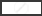t2 =t3 =,...
∴ a =d =Q 2 | Page 66

Decide whether following sequence is an A.P., if so find the 20th term of the progression.
–12, –5, 2, 9, 16, 23, 30,...

Q 3 | Page 66

Given Arithmetic Progression 12, 16, 20, 24, . . . Find the 24th term of this progression.

Q 4 | Page 66

Find the 19th term of the following A.P.

7, 13, 19, 25,....

Q 5 | Page 66

Find the 27th term of the following A.P.
9, 4, –1, –6, –11,...

Q 6 | Page 66

Find how many three digit natural numbers are divisible by 5.

Q 7 | Page 66

The 11th term and the 21st term of an A.P. are 16 and 29 respectively, then find the 41thterm of that A.P.

Q 8 | Page 66
11, 8, 5, 2,.... In this A.P. which term is number –151?
Q 9 | Page 66

In the natural numbers from 10 to 250, how many are divisible by 4?

Q 10 | Page 66

In an A.P. 17th term is 7 more than its 10th term. Find the common difference.

#### Chapter 3: Arithmetic Progression solutions [Pages 72 - 73]

Q 1 | Page 72
First term and common difference of an A.P. are 6 and 3 respectively ; find S27.
Q 2 | Page 72

Find the Sum of First 123 Even Natural Numbers.

Q 3 | Page 72
Find the sum of all even numbers from 1 to 350.
Q 4 | Page 72

In an A.P. 19th term is 52 and 38th term is 128, find sum of first 56 terms.

Q 5 | Page 72

Complete the following activity to find the sum of natural numbers from 1 to 140 which are divisible by 4.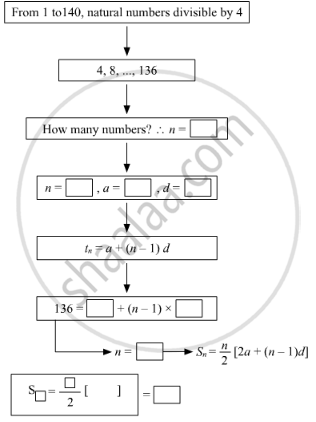Sum of numbers from 1 to 140, which are divisible by 4 =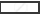Q 6 | Page 72

Sum of first 55 terms in an A.P. is 3300, find its 28th term.

Q 7 | Page 73

In an A.P. sum of three consecutive terms is 27 and their product is 504, find the terms.
( Assume that three consecutive terms in A.P. are a – d , a , a + d ).

Q 8 | Page 73

Find four consecutive terms in an A.P. whose sum is 12 and sum of 3rd and 4th term is 14.(Assume the four consecutive terms in A.P. are a – daa + da +2d)

Q 9 | Page 73

If the 9th term of an A.P. is zero then show that the 29th term is twice the 19th term ?

#### Chapter 3: Arithmetic Progression solutions [Page 78]

Q 1 | Page 78

On 1st Jan 2016, Sanika decides to save Rs 10, Rs 11 on second day, Rs 12 on third day. If she decides to save like this, then on 31st Dec 2016 what would be her total saving ?

Q 2 | Page 78

A man borrows Rs 8000 and agrees to repay with a total interest of Rs 1360 in 12 monthly instalments. Each instalment being less than the preceding one by Rs 40. Find the amount of the first and last instalment.

Q 3 | Page 78

Sachin invested in a national saving certificate scheme. In the first year he invested Rs 5000 , in the second year Rs 7000, in the third year Rs 9000 and so on. Find the total amount that he invested in 12 years.

Q 4 | Page 78

There is an auditorium with 27 rows of seats. There are 20 seats in the first row, 22 seats in the second row, 24 seats in the third row and so on. Find the number of seats in the 15th row and also find how many total seats are there in the auditorium ?

Q 5 | Page 78

Kargil’s temperature was recorded in a week from Monday to Saturday. All readings were in A.P. The sum of temperatures of Monday and Saturday was 5°C more than sum of temperatures of Tuesday and Saturday. If temperature of Wednesday was –30° celsius then find the temperature on the other five days.

Q 6 | Page 78

On the world environment day tree plantation programme was arranged on a land which is triangular in shape. Trees are planted such that in the first row there is one tree, in the second row there are two trees, in the third row three trees and so on. Find the total number of trees in the 25 rows.

#### Chapter 3: Arithmetic Progression solutions [Pages 78 - 80]

Q 1.01 | Page 78

Choose the correct alternative answer for  the following question .
The sequence –10, –6, –2, 2,...

(A) is an A.P.,Reason d = -16  (B) is an A.P Reason d = (C) is an A.P.,Reason d = -4 (D) is not an A.P.
Q 1.02 | Page 78

Choose the correct alternative answer for  the following question .

First four terms of an A.P. are ....., whose first term is –2 and common difference is –2.

(A) -2,0,2,4   (B) -2,4 ,-8,16

(C)-2, -4,-6,-8  (D) -2, -4 ,-8,-16

Q 1.03 | Page 78

Choose the correct alternative answer for  the following question .

What is the sum of the first 30 natural numbers ?

(A) 464  (B)465 (C)462 (D)461
Q 1.04 | Page 78

Choose the correct alternative answer for  the following question .

For an given A.P. t7 = 4, d = –4, n = 101, then a = ....

(A) 6 (B) 7 (C)  20 (D)  28

Q 1.05 | Page 78

Choose the correct alternative answer for  the following question .

For an given A.P. a = 3.5, d = 0, n = 101, then tn = ....

(A) 0  (B) 3.5  (C) 103.5 (D)14.5

Q 1.06 | Page 78

Choose the correct alternative answer for  the following question .

In an A.P. first two terms are –3, 4 then 21st term is ...

(A) -143   (B) 143  (C)  137  (D) 17

Q 1.07 | Page 78

Choose the correct alternative answer for  the following question .

If for any A.P. d = 5 then t18 – t13 = ....

(A) 5   (B) 20   (C) 25  (D) 30

Q 1.08 | Page 78

Choose the correct alternative answer for  the following question .

Sum of first five multuiples of 3 is...

(A) 45  (B) 55  (C) 15  (D) 75

Q 1.09 | Page 78

Choose the correct alternative answer for  the following question .

15, 10, 5,... In this A.P sum of first 10 terms is...

(A) -75 (B) -125 (C) 75 (D) 125

Q 1.1 | Page 78

Choose the correct alternative answer for  the following question .

In an A.P. 1st term is 1 and the last term is 20. The sum of all terms is = 399 then n = ....

(A) 42 (B) 38 (C) 21  (D) 19

Q 2 | Page 79

Find the fourth term from the end in an A.P. –11, –8, –5,...., 49.

Q 3 | Page 79

In an A.P. the 10th term is 46 sum of the 5th and 7th term is 52. Find the A.P.

Q 4 | Page 79

The A.P. in which 4th term is –15 and 9th term is –30. Find the sum of the first 10 numbers.

Q 5 | Page 79

Two A.P.’ s are given 9, 7, 5, . . . and 24, 21, 18, . . . . If nth term of both the progressions are equal then find the value of n and n th term.

Q 6 | Page 79

If sum of 3rd and 8th terms of an A.P. is 7 and sum of 7th and 14th terms is –3 then find the 10 th term .

Q 7 | Page 79

In an A.P. the first term is –5 and last term is 45. If sum of all numbers in the A.P. is 120, then how many terms are there ? What is the common difference ?

Q 8 | Page 79

Sum of 1 to n natural numbers is 36, then find the value of n .

Q 9 | Page 80

Divide 207 in three parts, such that all parts are in A.P. and product of two smaller parts will be 4623.

Q 10 | Page 80

There are 37 terms in an A.P., the sum of three terms placed exactly at the middle is 225 and the sum of last three terms is 429. Write the A.P.

Q 11 | Page 80

If first term of an A.P. is a, second term is b and last term is c, then show that sum of all terms is  $\frac{\left( a + c \right) \left( b + c - 2a \right)}{2\left( b - a \right)}$.

Q 12 | Page 80

If the sum of first p terms of an A.P. is equal to the sum of first q terms then show that the sum of its first (p + q) terms is zero. (p ≠ q)?

Q 13 | Page 80

If m times the mth term of an A.P. is eqaul to n times nth term then show that the (m + n)th term of the A.P. is zero.

Q 14 | Page 80

Rs 1000 is invested at 10 percent simple interest. Check at the end of every year if the total interest amount is in A.P. If this is an A.P. then find interest amount after 20 years. For this complete the following activity.

## Chapter 3: Arithmetic Progression

#### Balbharati Balbharati Class 10 Mathematics 1 Algebra## Balbharati solutions for Class 10th Board Exam Algebra chapter 3 - Arithmetic Progression

Balbharati solutions for Class 10th Board Exam chapter 3 (Arithmetic Progression) include all questions with solution and detail explanation. This will clear students doubts about any question and improve application skills while preparing for board exams. The detailed, step-by-step solutions will help you understand the concepts better and clear your confusions, if any. Shaalaa.com has the Maharashtra State Board Balbharati Class 10 Mathematics 1 Algebra solutions in a manner that help students grasp basic concepts better and faster.

Further, we at Shaalaa.com provide such solutions so that students can prepare for written exams. Balbharati textbook solutions can be a core help for self-study and acts as a perfect self-help guidance for students.

Concepts covered in Class 10th Board Exam Algebra chapter 3 Arithmetic Progression are Introduction to Sequence, Arithmetic Progression Examples and Solutions, Terms in a sequence, Geometric Mean, Arithmetic Progression, Geometric Progression, General Term of an Arithmetic Progression, General Term of an Geomatric Progression, Sum of First n Terms of an AP, Sum of the First 'N' Terms of an Geometric Progression, Arithmetic Mean.

Using Balbharati Class 10th Board Exam solutions Arithmetic Progression exercise by students are an easy way to prepare for the exams, as they involve solutions arranged chapter-wise also page wise. The questions involved in Balbharati Solutions are important questions that can be asked in the final exam. Maximum students of Maharashtra State Board Class 10th Board Exam prefer Balbharati Textbook Solutions to score more in exam.

Get the free view of chapter 3 Arithmetic Progression Class 10th Board Exam extra questions for and can use Shaalaa.com to keep it handy for your exam preparation

S